# The Lexicographic Construction

A q-ary, length n, minimum distance d lexicode is traditionally defined constructively based on a lexicographic (i.e., dictionary) ordering of vectors in which, for example, 01111 comes before 10000. The construction starts with the set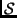= {0} and greedily adds, until exhaustion, the lexicographically earliest vector whose Hamming distance fromis at least d.

For example, the codewords of the binary lexicode (i.e., q = 2) of length n = 3 and minimum distance d = 2 are marked by ain Figureand would be computed left-to-right across the figure.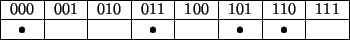This greedy construction always generates a linear code [3,5,9]. Thus, we may completely describe a dimension k lexicode by finding k basis vectors (known as generators'') using what we call the lexicographic construction. This construction starts with the zero code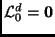and greedily adds the lexicographically earliest vector of distance d from the linear space spanned by the previously added vectors; k such iterations form the dimension k code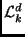. Table 1 demonstrates this construction for d = 3; the resulting code is a (7, 4, 3) binary code, meaning that it has length 7, dimension 4, and minimum distance 3.

Table 1: Generator matrix for the dimension 4, minimum distance 3 binary code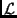43. The generator paddings are in bold.
 0000111 0011001 0101010 1001011

We may understand the lexicographic construction more analytically by making use of the covering radius of each intermediate code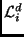in the iteration. The covering radius of a length n code is the smallest integer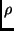with the property that Hamming balls of radiuscentered at codewords of the code will cover every vector of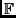qn. In other words,is the maximum distance of a vector inqn from the code. As an example, one can readily see that the binary lexicode in Figure 1 has a covering radius of 1 because every vector in23 is at most of Hamming distance 1 from a code vector.

An iteration of the lexicographic construction on an intermediate code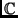whose covering radius isand minimum distance is d can thus be understood as the addition of a generator vector: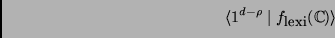where 1d -, known as the generator padding, has the usual meaning of (d -) successive 1's, and the functionreturns the lexicographically earliest vector of distancefrom: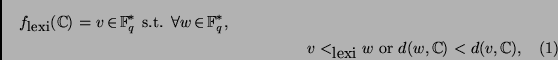where we define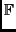q* =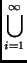qi.

We use the notation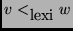to denote that v appears before w in a lexicographical ordering ofq*. Moreover we use the customary notation. | .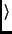to denote concatenation.

The linear codes generated by the lexicographic construction described above are precisely the lexicodes.

Theorem 1   Over a finite fieldq, a dimension k, minimum distance d codeis a lexicode if and only if it is produced by the lexicographic construction, namely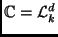.

Proof: We first prove thatkd is always a lexicode, by induction on k for an arbitrary, fixed d. For the base case it is clear that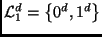is a (d, 1, d ) lexicode. Now assume as an inductive hypothesis thatkd is a dimension k, minimum distance d lexicode. From the definition of the lexicographic construction,k + 1d has parameters (n + d -, k + 1, d ), whereis the covering radius ofkd. Consider the (n + d -, k', d ) lexicode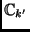constructed by repeatedly choosing the appropriate lexicographically-earliest vectors inqn + d -. Clearly, we generate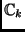in the process of this construction, so thatand, by the inductive hypothesis,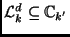. Moreover, the vector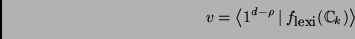is the lexicographically earliest vector of distancefrom. Thus, it must be the case that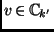and, sinceis linear,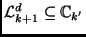.

In addition, any vector v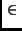qn + d -that is inbut not ink + 1d would necessarily have its n right-most bits at distance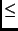fromk + 1d, and its other bits at distance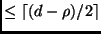fromk + 1d. Noting that< d, we may now use the triangle inequality to see that the distance from v tok + 1d (and consequently also) must be at most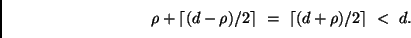This contradicts our constructive definition of, so it must be that.

For the converse assertion of the theorem, we need to show that a non-trivial (n, k, d ) lexicodecan be constructed by the lexicographic construction. The codekd produced by k iterations of the minimum-distance d lexicographic construction is clearly a lexicode, from the first part of the proof. Since all distance d lexicodes are ordered by inclusion, as we saw in the first part of this proof, we may conclude that eitheror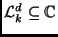. Howeverandare both non-trivial5 dimension k codes, so it follows that they must be equal.

width4pt depth2pt height6pt

Theorem 1 allows us to bypass the codeword-by-codeword lexicode construction, and instead directly compute the generator matrix of a desired lexicode.

Subsections